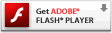# Interactive Generative Art Series – 02 – Random ColorWith this example we’re taking a look at having randomly changing color. I liked the limits to the color in the last post, but couldn’t help myself and wanted to see it with the full range of colors to command. Also, I wasn’t a huge fan when the color would “wrap” and jump from one color to the next so fast. I wanted to use the same velocity (rate of change) principle from the line width experiment but apply it to color, and have it meander aimlessly among all colors. I created a color object to store a value for each of the RGB color values, and then had each element of the color change independently. I didn’t want to complicate my loop function so I made a new function that is called from loop that steps along to a color which should be pretty close to the previous color. I enjoy the range, but as I expected it was a bit much, or too many colors at once, also the step is a bit too fast at times it seems.

### 02 Color, play here

Please visit the blog article to view this interactive flash content. Flash plug-in required:### actionscript source code

[cc lang=”actionscript”]
var ball:Sprite = new Sprite();

ball.graphics.beginFill(0x333333, 1);
ball.graphics.drawCircle(0, 0, 30);
ball.graphics.endFill();

var div:Number = .1;

var ax:Number = 0;
var ay:Number = 0;

var line_max_width:Number = 50;
var line_min_width:Number = 0;
var line_width:Number = Math.random() * line_max_width;
var line_width_velocity:Number = 0;
var dampen:Number = 0.95;

var colors:Object = new Object();
colors.r = 0;
colors.g = 0;
colors.b = 255;
colors.rv = 0;
colors.gv = 0;
colors.bv = 0;

function loop () {
ball.x -= ax = (ax + (ball.x – mouseX) * div) * .9;
ball.y -= ay = (ay + (ball.y – mouseY) * div) * .9;

line_width_velocity += Math.random() * 6 – 3;
line_width += line_width_velocity;
line_width_velocity *= dampen;
if(line_width > line_max_width) { line_width = line_max_width; }
if (line_width < line_min_width) { line_width = line_min_width; }

color_step();

this.graphics.lineStyle(line_width, rgb2hex(colors.r, colors.g, colors.b), 1);
this.graphics.lineTo(ball.x, ball.y);
}

setInterval(loop, 1000/30);

function rgb2hex(r:Number, g:Number, b:Number):Number {
return(r<<16 | g<<8 | b); } function color_step(){ colors.rv += Math.random() * 20 – 10; colors.r += colors.rv; colors.rv *= dampen; if (colors.r > 255) {
colors.r = 255;
} else if (colors.r < 0){ colors.r = 0; } colors.gv += Math.random() * 20 – 10; colors.g += colors.gv; colors.gv *= dampen; if (colors.g > 255) {
colors.g = 255;
} else if (colors.g < 0){ colors.g = 0; } colors.bv += Math.random() * 20 – 10; colors.b += colors.bv; colors.bv *= dampen; if (colors.b > 255) {
colors.b = 255;
} else if (colors.b < 0){
colors.b = 0;
}
}
[/cc]